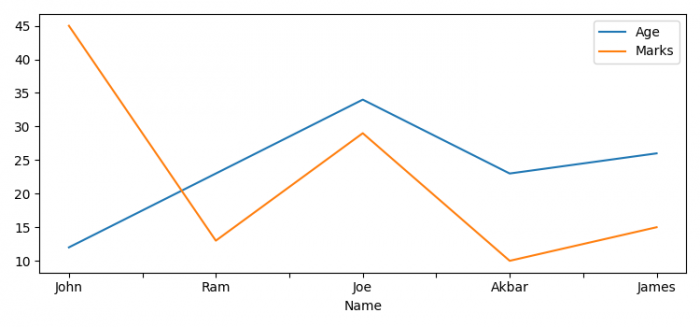# How to plot CSV data using Matplotlib and Pandas in Python?

To plot CSV data using Matplotlib and Pandas in Python, we can take the following steps −

• Set the figure size and adjust the padding between and around the subplots.
• Make a list of headers of the .CSV file.
• Set the index and plot the dataframe.
• To display the figure, use show() method.

## Example

import pandas as pd
import matplotlib.pyplot as plt

plt.rcParams["figure.figsize"] = [7.50, 3.50]
plt.rcParams["figure.autolayout"] = True

plt.show()